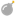## FANDOM

22,194 Pages

Magnitude is a term used across mathematics that means several things. Magnitude can also been seen as a "Size" of something, the magnitude of anExplosion is the size of the explosion. Because the magnitude is the size of something it can't be negative.

The magnitude of a real number a is |a| (also seen as math.abs(a)), this means that the '-' of the value will always be removed.

```print(math.abs(10)) -- Prints '10'
print(math.abs(-10)) -- Prints '10'
print(-math.abs(10)) -- Prints '-10'
print(-math.abs(-10)) -- Prints '-10'
```

But for complex numbers it is a bit more complicated. A complex number exist of two values, the real part and the imaginary part (a + bi). An example of an complex value is 4 + 10i. To get the magnitude of a complex value you do: √(a²+b²). You say that if Z = 4 + 10i, then |Z| = √(4²+10²).

In vector theory, the magnitude of a vector is the length of a vector. An example use in script:

```Position1 = Vector3.new(0,0,0)
Position2 = Vector3.new(0,0,10)
print((Position1-Position2).magnitude) -- Prints '10'
```

This is used to get the length between two positions.

Community content is available under CC-BY-SA unless otherwise noted.